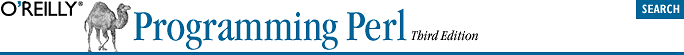home | O'Reilly's CD bookshelfs | FreeBSD | Linux | Cisco | Cisco Exam## 9.3. Arrays of Hashes

An array of hashes is useful when you have a bunch of records that you'd like to access sequentially, and each record itself contains key/value pairs. Arrays of hashes are used less frequently than the other structures in this chapter.

### 9.3.1. Composition of an Array of Hashes

You can create an array of anonymous hashes as follows:

```@AoH = (
{
husband  => "barney",
wife     => "betty",
son      => "bamm bamm",
},
{
husband => "george",
wife    => "jane",
son     => "elroy",
},

{
husband => "homer",
wife    => "marge",
son     => "bart",
},
);```
To add another hash to the array, you can simply say:
`push @AoH, { husband => "fred", wife => "wilma", daughter => "pebbles" };`

### 9.3.2. Generation of an Array of Hashes

Here are some techniques for populating an array of hashes. To read from a file with the following format:

`husband=fred friend=barney`
you could use either of the following two loops:
```while ( <> ) {
\$rec = {};
for \$field ( split ) {
(\$key, \$value) = split /=/, \$field;
\$rec->{\$key} = \$value;
}
push @AoH, \$rec;
}

while ( <> ) {
push @AoH, { split /[\s=]+/ };
}```
If you have a subroutine get_next_pair that returns key/value pairs, you can use it to stuff @AoH with either of these two loops:
```while ( @fields = get_next_pair() ) {
push @AoH, { @fields };
}

while (<>) {
push @AoH, { get_next_pair(\$_) };
}```
You can append new members to an existing hash like so:
```\$AoH{pet} = "dino";
\$AoH{pet} = "santa's little helper";```

### 9.3.3. Access and Printing of an Array of Hashes

You can set a key/value pair of a particular hash as follows:

`\$AoH{husband} = "fred";`
To capitalize the husband of the second array, apply a substitution:
`\$AoH{husband} =~ s/(\w)/\u\$1/;`
You can print all of the data as follows:
```for \$href ( @AoH ) {
print "{ ";
for \$role ( keys %\$href ) {
print "\$role=\$href->{\$role} ";
}
print "}\n";
}```
and with indices:
```for \$i ( 0 .. \$#AoH ) {
print "\$i is { ";
for \$role ( keys %{ \$AoH[\$i] } ) {
print "\$role=\$AoH[\$i]{\$role} ";
}
print "}\n";
}```